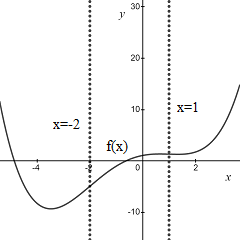# Sketch the graph for a single function that has all of the properties listed. f''(x) less than 0...

## Question:

Sketch the graph for a single function that has all of the properties listed.

{eq}f''(x) < 0 {/eq} on {eq}(-2, 1) {/eq}

## Concavity of a Function:

A function of one real variable f(x) is concave up in the intervals where its second derivative is positive.

The function is concave down where its second derivative is negative.

The inflection points are those points where the second derivative is zero.

We know that a function f(x) is concave down on the interval (-2,1). Then, its second

derivative is negative over such interval {eq}f''(x) < 0 {/eq}.

Since the function has inflection points at {eq}x=-2 {/eq} and {eq}x=1 {/eq}

we search a function with a second derivative given by

{eq}f''(x) = (x+2)(x-1) = x^2 +x -2. {/eq}

The function f(x) is found by integrating twice its second derivative

{eq}\displaystyle f'(x) = \frac{1}{3}x^3 +\frac{1}{2}x^2 -2x + C \\ \displaystyle f(x) = \frac{1}{12}x^4 +\frac{1}{6}x^3 - x^2 + Cx + D. {/eq}

The function obtained when setting {eq}C=D=1 {/eq}, that is

{eq}\displaystyle f(x) = \frac{1}{12}x^4 +\frac{1}{6}x^3 - x^2 + x + 1 {/eq}

is illustrated in the figuer below.